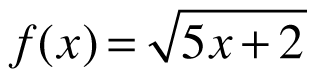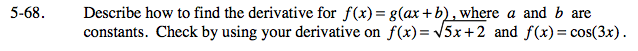### Home > CALC > Chapter 5 > Lesson 5.2.2 > Problem5-68

5-68.

Describe how to find the derivative for f(x) = g(ax + b), where a and b are constants. Check by using your derivative onand f(x) = cos(3x). Homework Help ✎Both of these functions are composites and require the Chain Rule to derive. Start by comparing each function to f(x) + g(ax + b).
Then explain how the Chain Rule works.